# Solutions to Mathathon

I LOVE THE DREAM SMP!
There are many ways to look at this problem. Three good ways would be:

## 1. Directly calculate if you know the formula for frustum volume.

The formula for frustum volume, given bottom radius $R$, top radius $r$ and height $h$, is $V=\frac{1}{3}\pi h(R^2+r^2+Rr).$ Therefore we can plug in $\pi\approx \frac{22}{7}, ~~h=70,~~R=40,~~r=70$ to find the volume (must be converted to Liters): $V=\frac{1}{3}\times \frac{22}{7}\times 70(40^2+70^2+40\times 70=682000 (\text{cm}^3)=\color{#D61F06}682 \text{L}.$

## 2. Calculate difference of cones not knowing the formula for frustum volume.

Extending the cone by imagination and using similarity, we get the pic below: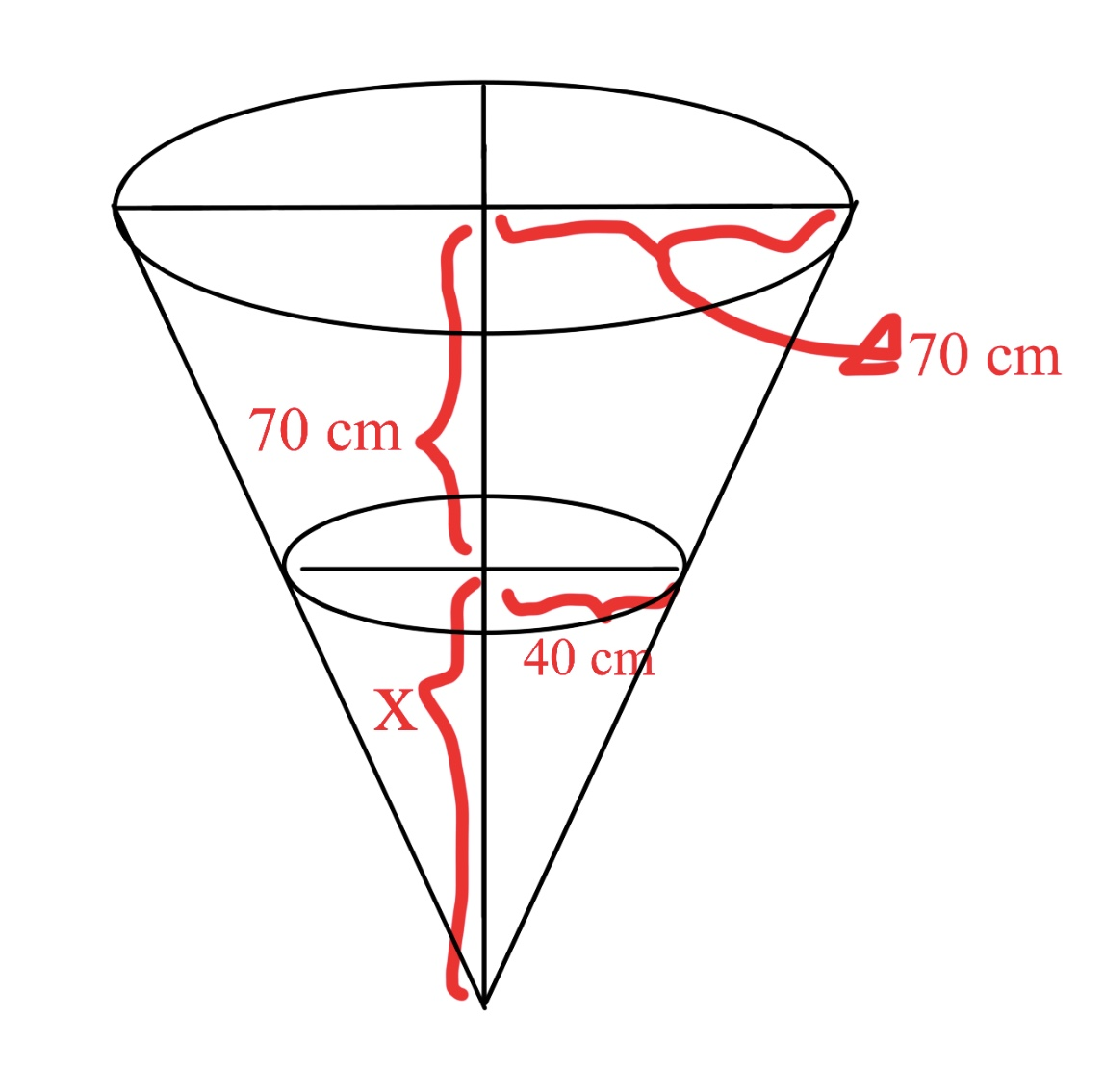Let the height of the increased cone be $x$. By similarity, we get $\frac{40}{x}=\frac{70}{70+x}$. Solve this to get $x=\frac{280}{3}$.
So the volume of the frustum is the volume of the larger cone minus the volume of the smaller cone (the formula for cone volume is $\frac{1}{3}\pi r^2h$ where $r$ stands for bottom radius and $h$ stands for height): $V_{\text{frustum}}=V_{\text{big cone}}-V_{\text{small cone}}=\frac{1}{3}\pi \times 70^2\times (70+\frac{280}{3})-\frac{1}{3}\pi \times 40^2\times \dfrac{280}{3}=682000(\text{cm}^3)~~~\color{#D61F06}\pi~\text{is replaced with}~\frac{1}{3}~\text{here}$

## 3. Use the ‘Baumkuchen’ integral.

$\scriptsize \color{#D61F06} \text{If under 18, do NOT do at home without adult supervision to avoid brain explosion.}$
This is mentioned in a book I read which finds volumes of 3D-shapes created by rotating the shape bounded by functions $f(x)$ and $g(x)$ in region $a\le x\le b$: $V=2\pi \int_a^b|x||f(x)-g(x)| dx.$ The absolute value brackets are there to make sure $V$ is positive. THIS WORKS ONLY IF THE ROTATED BOUND IS ON THE SAME SIDE OF THE Y-AXIS.
Why is it called Baumkuchen?
It is because Baumkuchen is ‘log-like dessert’ in German, and the integral is like one!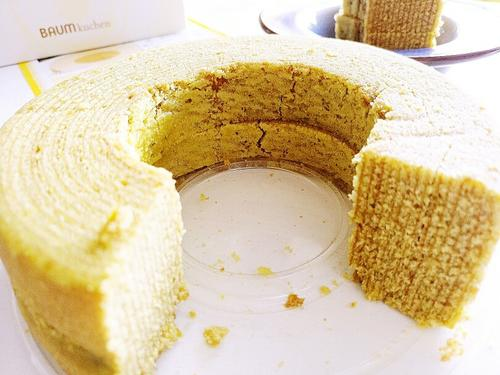Baumkuchen has log-like stripes Explanation:
We can split the integral into rings formed by the original bound split and rotated around the y-axis individually.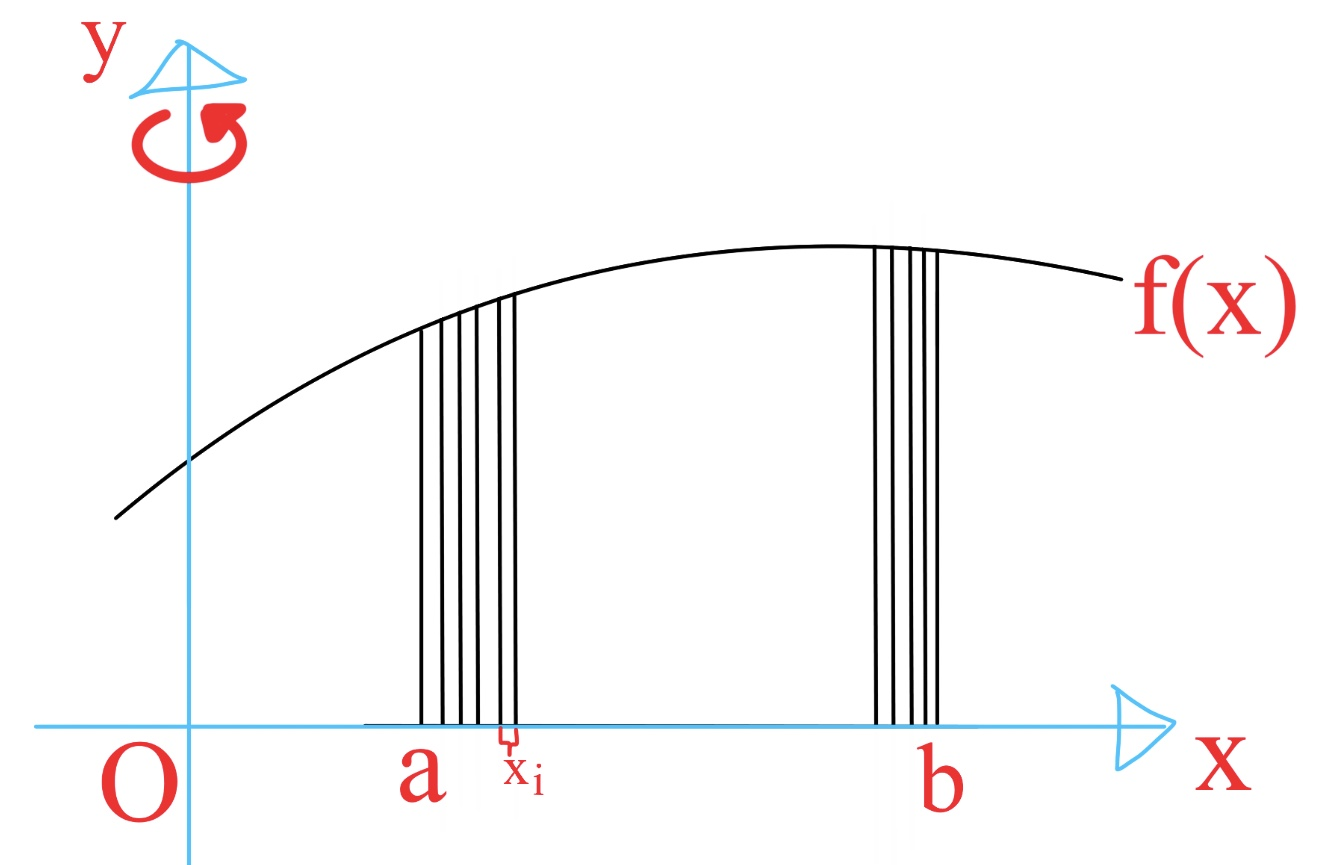Here I made a little mistake when labelling :P $x_i$ should be replaced with $dx$ :)
For simplicity, here I let $g(x)=0$. Of course this can be generalised to other functions as well, given above.
If we cut a single ‘ring’ open, we get its volume by seeing it as a cuboid: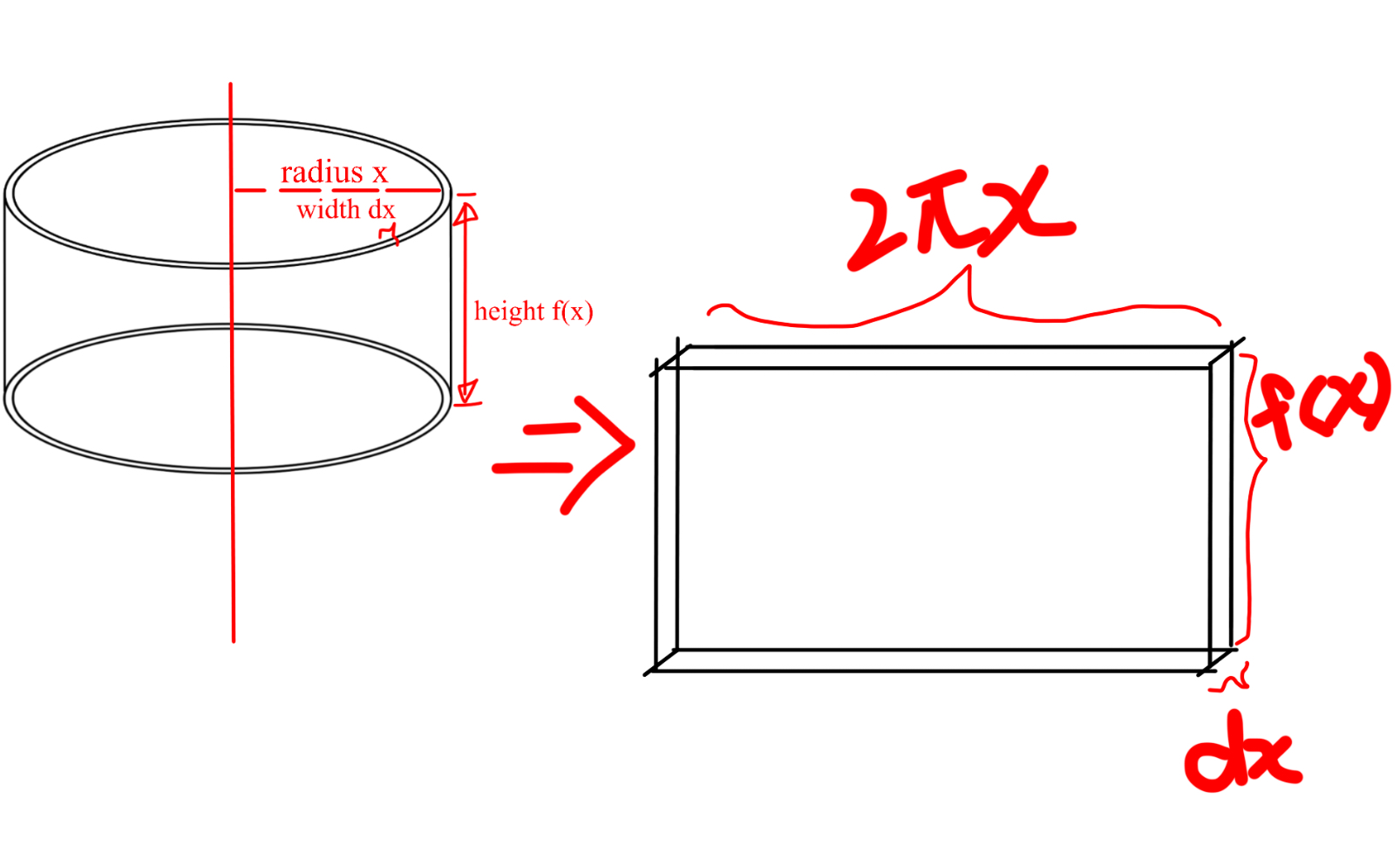I forgot the absolute value brackets :P Enlarge to see clearer :)
Here the volume of the cuboid is $2\pi |x||f(x)|$ because $g(x)=0$ and the $-g(x)$ term is therefore neglected. Summing infinite cuboids gives $V=2\pi \int_a^b|x||f(x)-g(x)| dx.$ So we can see the frustum as a cone chopped off from another as in example 2. This way, the volume of the big cone is the integral with $a=0,b=70$, $f(x)=70$ and $g(x)=\frac{7}{3}x-\frac{280}{3}$.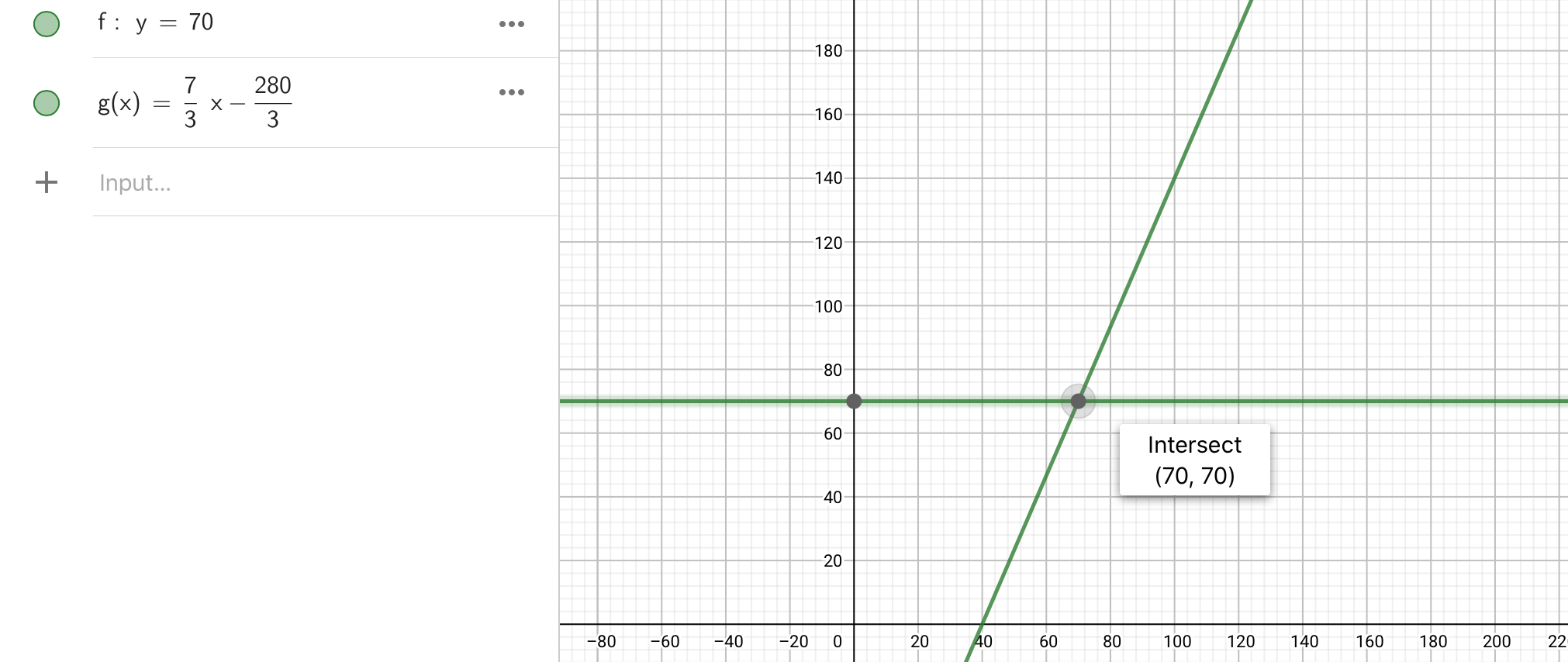Plug in these to get $V_1=2\pi\int_0^{70} |x||70-(\frac73x-\frac{280}{3})=2\pi \int_0^{70} \frac{490}{3}x-\frac{7}{3}x^2\approx 2\times\frac{22}{7}(\left. \frac{245}{3}x^2-\frac79x^3\right|_0^{70})=75460009.$ Similarly we can apply the same to the small cone to get $V_2=\frac{1894000}{9}$. $V_1-V_2=628000.$ Convert to liters: $628$.Note by Jeff Giff
4 months ago

This discussion board is a place to discuss our Daily Challenges and the math and science related to those challenges. Explanations are more than just a solution — they should explain the steps and thinking strategies that you used to obtain the solution. Comments should further the discussion of math and science.

When posting on Brilliant:

• Use the emojis to react to an explanation, whether you're congratulating a job well done , or just really confused .
• Ask specific questions about the challenge or the steps in somebody's explanation. Well-posed questions can add a lot to the discussion, but posting "I don't understand!" doesn't help anyone.
• Try to contribute something new to the discussion, whether it is an extension, generalization or other idea related to the challenge.

MarkdownAppears as
*italics* or _italics_ italics
**bold** or __bold__ bold
- bulleted- list
• bulleted
• list
1. numbered2. list
1. numbered
2. list
Note: you must add a full line of space before and after lists for them to show up correctly
paragraph 1paragraph 2

paragraph 1

paragraph 2

[example link](https://brilliant.org)example link
> This is a quote
This is a quote
    # I indented these lines
# 4 spaces, and now they show
# up as a code block.

print "hello world"
# I indented these lines
# 4 spaces, and now they show
# up as a code block.

print "hello world"
MathAppears as
Remember to wrap math in $$ ... $$ or $ ... $ to ensure proper formatting.
2 \times 3 $2 \times 3$
2^{34} $2^{34}$
a_{i-1} $a_{i-1}$
\frac{2}{3} $\frac{2}{3}$
\sqrt{2} $\sqrt{2}$
\sum_{i=1}^3 $\sum_{i=1}^3$
\sin \theta $\sin \theta$
\boxed{123} $\boxed{123}$

Sort by:

You are 13 and u know integration? Now that's CoOoOOOoOlll!

- 3 months, 3 weeks ago

Oh uhh yeaaah :)

- 3 months, 3 weeks ago

U know what I’m actually making a calculus note to help unsubscribed users like me :)

- 3 months, 3 weeks ago

- 3 months, 3 weeks ago

# Great job !

- 3 months, 3 weeks ago

- 3 months, 2 weeks ago

It’s actually precisely translated I should say :) but I can comprehend English well enough without the help of translators anyway :)

- 3 months, 2 weeks ago

I wanted to sound CoOoOOOoOlll that's why I did that, not bcz I was doubtful :D

- 3 months, 2 weeks ago

Besides you might like to know sir Zakir Husain, he sometimes needs help with solving stuff with programs :)

- 3 months, 2 weeks ago

Yeah sure:)

- 3 months, 2 weeks ago

You know what?
1. I joined brilliant one year ago, when I lacked at calculus :P (Besides brilliant doesn’t allow me to chose 12-year-old! I changed it back on my birthday)
2. You’re the only one to realise my age
3. I am the youngest brilliant user known for the time being
4. I feel like Sheldon

- 3 months, 3 weeks ago

1.Haha what a fun story ,the best part was u telling that u were not so good in calculus at the time when I didn't even know what the heck it is XP.

2.Are u serious?I mean it's mentioned in almost all the ques posted by u¯\(ツ)/¯.

3.I didn't wanna say it but.....

# Aww

.4. Haha ,weird flex but okay.. [face-palm emoji] ᕙ( • ‿ • )ᕗ

- 3 months, 3 weeks ago

Haha :D

- 3 months, 2 weeks ago

Well, people have to go to my profile to see my age so yeah, hardly anyone knows!

- 3 months, 2 weeks ago

Actually some dude in America does calculus better than me (screw it! :P) and he’s only 15 :D besides integration always gives me a headache (goddamn calculus fries my brain every time ¯(ツ)/¯)

- 3 months, 2 weeks ago

Haha it's okay,you will get the essence of it with lots and lots of practice.Good luck:)

- 3 months, 2 weeks ago

Now coming to think of it... I suck at physics. Perhaps mastering physics will be my goal after mastering calculus :)

- 3 months, 2 weeks ago

I loOoOve physics,ask me anything and I'll spill all the facts about that topic:D

- 3 months, 2 weeks ago